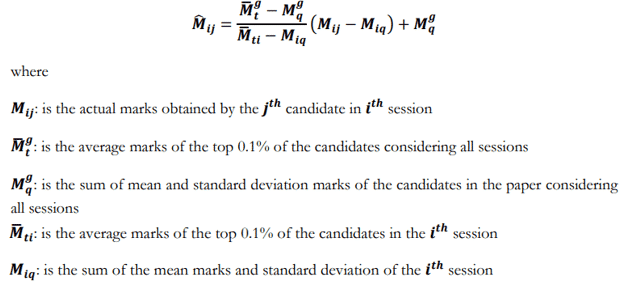# GATE 2021 Score Card Calculation (Out Soon), Normalization Process – Check Authentic Formula hereThe Scorecard will be available to those candidates who will score higher than the scores set by the GATE 2021 Committee. Normalization scores will be calculated for the examinations conducted. in multiple sessions. The GATE 2021 committee is the authority to decide the qualification/pass mark for each GATE document.

Detailed information on calculating the GATE 2021 dashboard is provided below. Applicants have many questions related to the GATE 2021 Normalization Process. Applicants are excited to know the Process to calculate their score secured by them in the GATE 2021 Entrance Examination.  The GATE Normalization Process is a method to change applicants’ Rough Marks into the Scores after computing the rough marks into the Score.

25 Papers are conducted in the GATE in which some papers are held in multiple sessions as per the number of candidates. For the single session and the multiple session, there will be a different Normalization Process for it. In the case of the single session, rough marks will be converted into a score by the computing method. For the multiple session, there will be exact marks will be converted into GATE Score.

## Difference between GATE Marks and GATE Score

GATE 2021 Entrance Examination contains a Total of 100 Marks Questions. The GATE marks are the actual marks that are scored by the candidates out of 100. After Normalizing the GATE Marks, it will be termed as GATE Score. The maximum gate score is 1000.

However, the candidate who has scored 1000 does not mean candidates have 100 Marks in GATE 2021. The marks after calculation could be 1000, as the consideration and calculation are done on various factors.

## Calculation of GATE Score (CE/CS/EC/EE/ME)

The number of candidates for CE, CS, EC, EE and ME is important, therefore, the GATE 2021 Examination will be done in several sessions.

Therefore, the committee decided to calculate the standardized marks separately for the GATE 2021 Examination with the paper codes CE, CS, EC, EE and ME. After considering several factors and standardization formulas, the following formula is designed to calculate standardized marks:

## Is there a Qualifying Cutoff marks in GATE and what is it?Normalized Mark of the jth candidate in ith session M^ it is as follows:

M^ij=Ṁgt−Mgq/Mˉti−Miq(Mij−Miq)+Mgq

Here,

• Mij is the actual marks obtained by the jth candidate in ith session
• Mˉgt is the average marks of the top 0.1% of the candidates considering all sessions
• Mg is the sum of mean and standard deviation marks of the candidates in the paper considering all session
• Mˉti is the average marks of the top 0.1% of the candidates in the ith session
• Miq is the sum of the mean marks and standard deviation of the ith session

With the help of the previous formula, the candidate’s gross marks will become standardized brands. Now, the candidate’s GATE score will be calculated based on the standard scores.

## Check Below Others Details –

GATE 2021 Cut Off

GATE 2021 Counseling

GATE 2021 Score Card

## Calculation of GATE Score (all Papers)

Candidates with the paper code other than CE, CS, EC, EE and ME must provide their actual scores for the GATE score calculation. The GATE 2021 score for all items will be evaluated by the following formula:GATE Score = Sq+(St−Sq)(M−Mq)(Mˉt−Mq)

Where,

• M= marks obtained by the candidate (actual marks for single session papers and normalized marks for multi-session papers).
• Mq = is the qualifying marks for general category candidate in the paper.
• Mˉt = is the mean of marks of top 0.1% or top 10 (whichever is larger) of the candidates who appeared in the paper (in case of multi-session papers including all sessions).
• Sq= 350, is the score assigned to Mq.
• St= 900, is the score assigned to Mˉt.

In the formula of GATE 2021 Score, Mq is generally 25 marks (out of 100) or μ+σ, whichever is larger. Here μ is the mean and σ is the standard deviation of the scores of all candidates who will appear on the GATE 2021 exam.

In case of any query or doubt regarding GATE 2021 Score Card Calculation, you may ask us by commenting below.